# 【装饰博客】一篇就够了

前言：虽说博客主要用来学习分享技术，但在学习之余能把自己的博客打扮的更独特，那是不错的选择。

• 申请博客的 js 权限（必须）；
• 博客背景上漂浮的磁力线，鼠标吸铁石；
• 鼠标点击出漂浮出字体("富强", "民主", "文明"...)；
• 在个人博客中播放自己喜欢的歌曲；
• 博客不使用 js 权限，也可以使用 js 的黑科技；

博客中为了安全考虑，默认是不可以进行 JS 编辑的，所以我们首先需要申请博客的 JS 权限。先进入 【管理】-【设置】 界面中；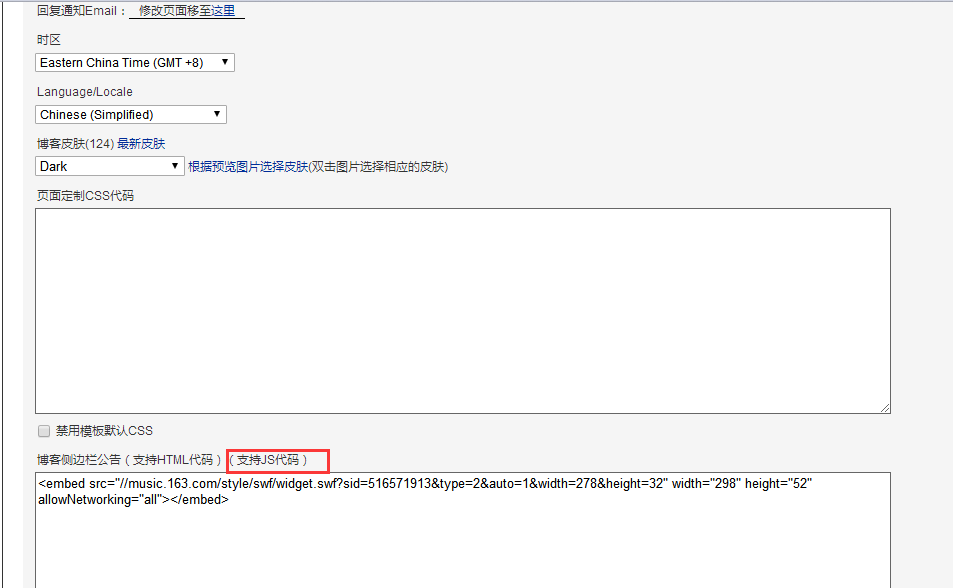在上面图中 红框 的位置会有申请 JS 代码权限的链接，进入填写你的申请原因，进行申请即可，我提交申请 大概一个小时之后审批通过了。

如果通过后就可以对博客进行美化了，如果没通过.....

你可能在很多博客中看到类似 当鼠标移动上去的时候会出现线条吸附在鼠标周围的特效，鼠标离开的时候线条就自动散开。（我的博客中就能看到）

这些特效都是调用 JS 来完成的。我贴出代码：

<script>

!function(){

function n(n,e,t){

return n.getAttribute(e)||t

}

function e(n){

return document.getElementsByTagName(n)

}

function t(){

var t=e("script"),o=t.length,i=t[o-1];

return{

l:o,z:n(i,"zIndex",-1),o:n(i,"opacity",.6),c:n(i,"color","255,255,255"),n:n(i,"count",150)　　<!-- opacity 参数设置的是透明程度，数字越小越透明；  color 设置颜色； count 设置磁线的数量  -->

}

}

function o(){

a=m.width=window.innerWidth||document.documentElement.clientWidth||document.body.clientWidth,

c=m.height=window.innerHeight||document.documentElement.clientHeight||document.body.clientHeight

}

function i(){

r.clearRect(0,0,a,c);

var n,e,t,o,m,l;

s.forEach(function(i,x){

for(i.x+=i.xa,i.y+=i.ya,i.xa*=i.x>a||i.x<0?-1:1,i.ya*=i.y>c||i.y<0?-1:1,r.fillRect(i.x-.5,i.y-.5,1,1),e=x+1;e<u.length;e++)n=u[e],

null!==n.x&&null!==n.y&&(o=i.x-n.x,m=i.y-n.y,

l=o*o+m*m,l<n.max&&(n===y&&l>=n.max/2&&(i.x-=.03*o,i.y-=.03*m),

t=(n.max-l)/n.max,r.beginPath(),r.lineWidth=t/2,r.strokeStyle="rgba("+d.c+","+(t+.2)+")",r.moveTo(i.x,i.y),r.lineTo(n.x,n.y),r.stroke()))

}),

x(i)

}

var a,c,u,m=document.createElement("canvas"),

d=t(),l="c_n"+d.l,r=m.getContext("2d"),

x=window.requestAnimationFrame||window.webkitRequestAnimationFrame||window.mozRequestAnimationFrame||window.oRequestAnimationFrame||window.msRequestAnimationFrame||

function(n){

window.setTimeout(n,1e3/45)

},

w=Math.random,y={x:null,y:null,max:2e4};m.id=l,m.style.cssText="position:fixed;top:0;left:0;z-index:"+d.z+";opacity:"+d.o,e("body").appendChild(m),o(),window.onresize=o,

window.onmousemove=function(n){

n=n||window.event,y.x=n.clientX,y.y=n.clientY

},

window.onmouseout=function(){

y.x=null,y.y=null

};

for(var s=[],f=0;d.n>f;f++){

var h=w()*a,g=w()*c,v=2*w()-1,p=2*w()-1;s.push({x:h,y:g,xa:v,ya:p,max:6e3})

}

u=s.concat([y]),

setTimeout(function(){i()},100)

}();

</script>

以上代码中 红色 注释是主要可以修改的参数了，你要是喜欢的话，还可以仔细研究研究。

这时，我们要做的就是把这段代码复制到 以下位置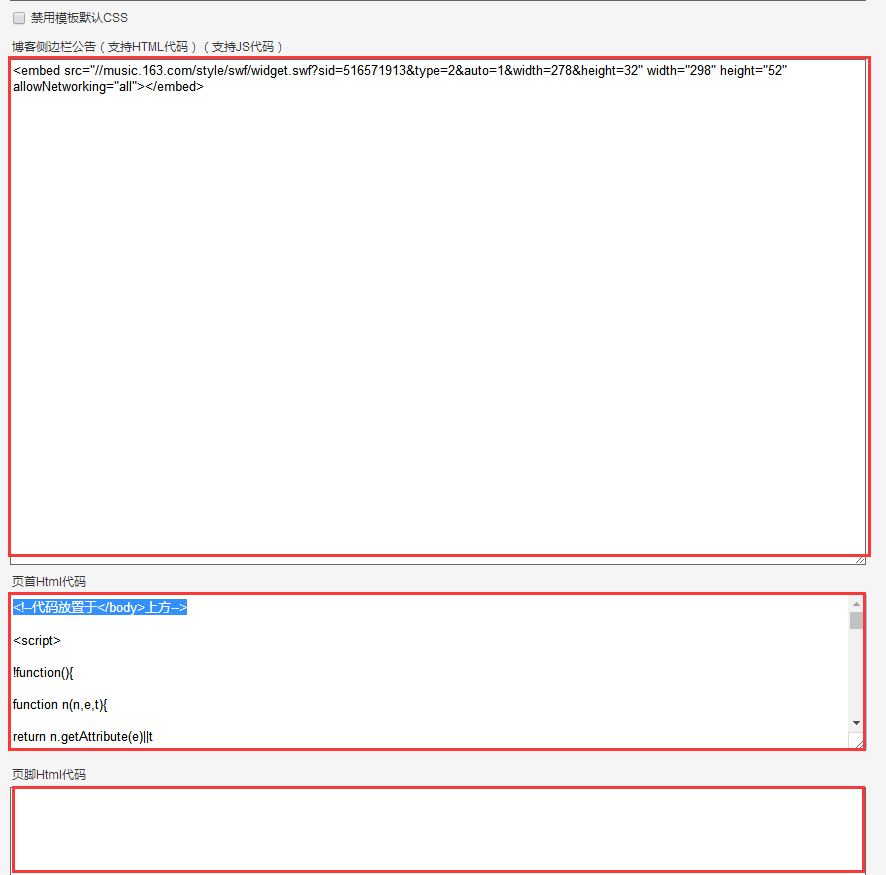这三个位置，就如介绍，分别是 博客侧边栏公告、页首、页脚。我们这里的 JS 代码放在哪里都是可以的。保存之后就能看到博客中出现效果了。

和上面一样，这个特效只需要复制 JS 代码就可以。贴代码

<script type="text/javascript">
/* 鼠标特效 */
var a_idx = 0;
jQuery(document).ready(function($) {$("body").click(function(e) {
var a = new Array("富强", "民主", "文明", "和谐", "自由", "平等", "公正" ,"法治", "爱国", "敬业", "诚信", "友善");　　　　　　/* 可想而知，这里可以修改显示的字 */
var $i =$("<span />").text(a[a_idx]);
a_idx = (a_idx + 1) % a.length;
var x = e.pageX,
y = e.pageY;
$i.css({ "z-index": 999999999999999999999999999999999999999999999999999999999999999999999, "top": y - 20, "left": x, "position": "absolute", "font-weight": "bold", "color": "#ff6651" });$("body").append($i);$i.animate({
"top": y - 180,
"opacity": 0
},
1500,
function() {
\$i.remove();
});
});
});
</script>

贴代码 保存之后就能看到博客中出现效果了。

其实，可以自己写 JS 代码，实现你自己喜欢的特效，做出独一无二的博客。

首先我们要做的就是进入 【网易云音乐网页版】，选择自己喜欢的歌曲。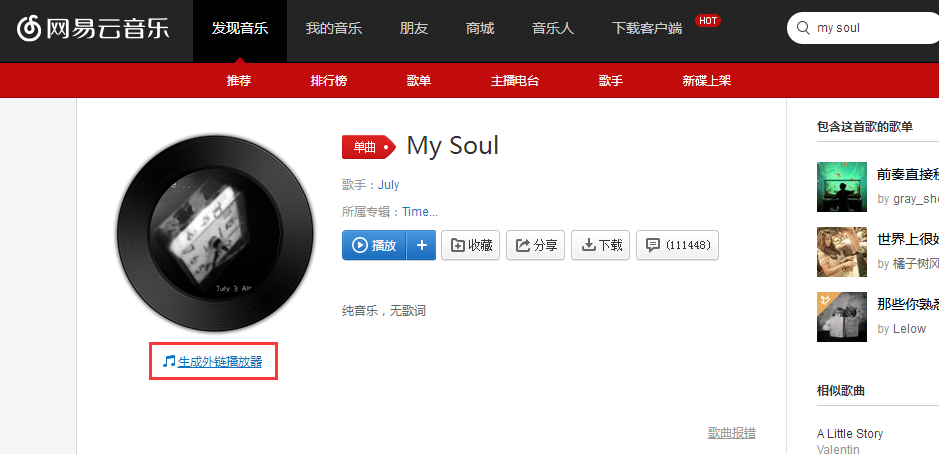点击 【生成外链播放器】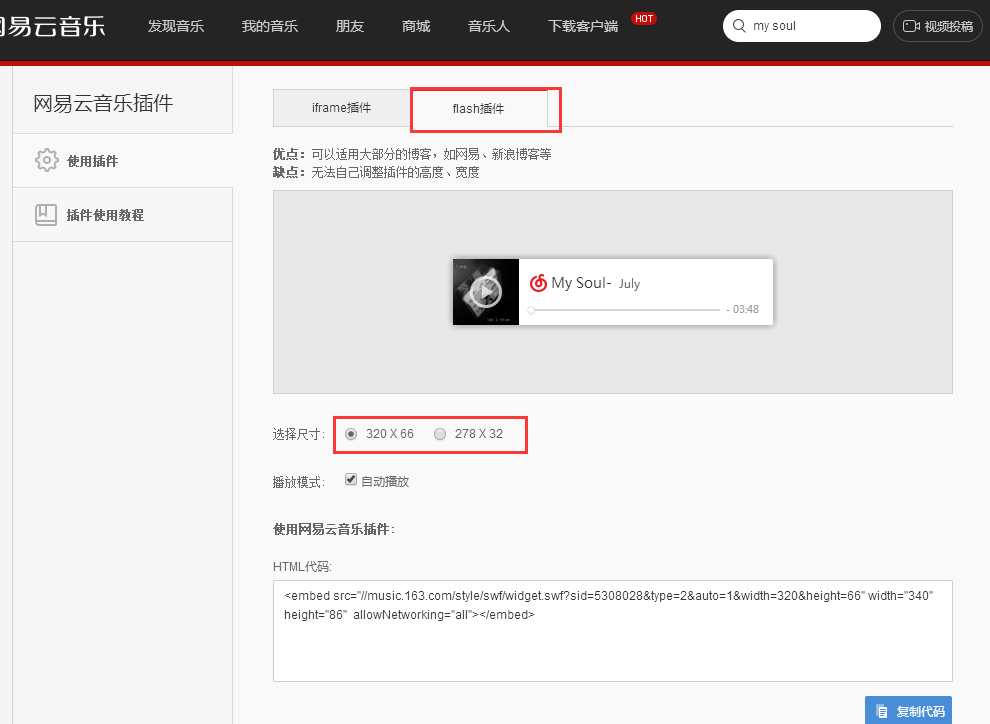这个地方需要注意的是，博客园不支持 iframe 插件，所以我们选择 flash 插件  ，再选择合适的尺寸。 复制 HTML 代码，把代码粘贴到 博客侧边栏公告。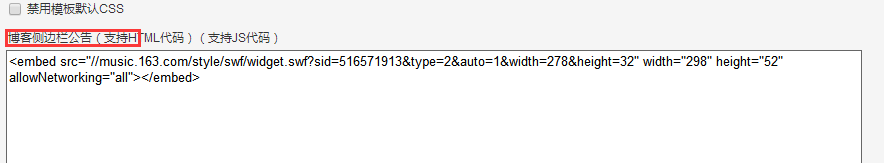保存后会在公告栏处出现 播放器。（当然了，如果歌曲太过另类，可能会吓跑访客，并且有些同学比较喜欢安静，所以谨慎考虑使用）

如果你没有获得 JS 权限的话，你再 页首HTML编辑的地方 使用 <script> 保存时会被删掉。

解决方法就是使用 <img> 标签。

<img src="." onerror="confirm('可以执行JS！')" />

在这里，给一个img标签编写一个错误的地址，这样会触发img标签的行内onerror事件，就可以执行任意JS代码了。

我们把这段代码，放到 页首HTML 中看看效果。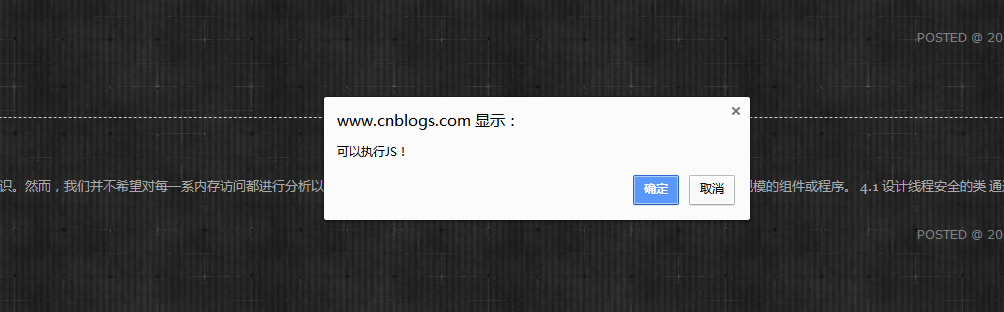只要找到了开口就可以利用此开口执行任何代码了。

posted @ 2018-08-02 14:50  躲猫猫的猫  阅读(696)  评论(1编辑  收藏  举报